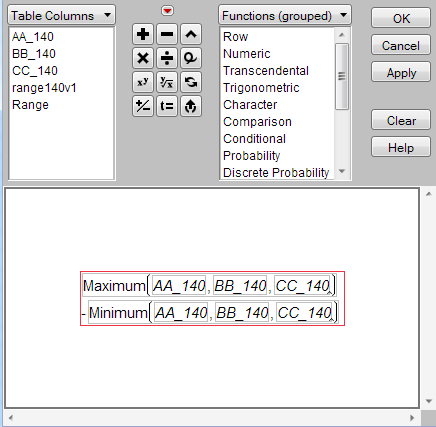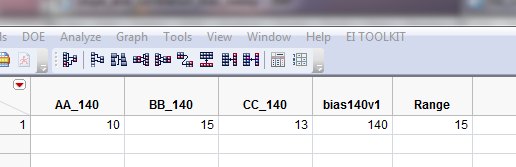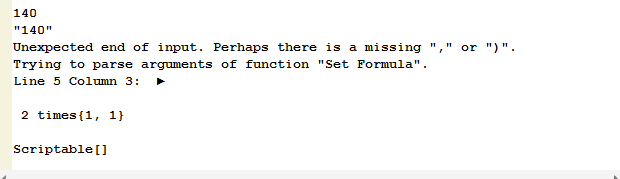BookmarkSubscribe
Choose Language Hide Translation Bar

Can I use a concatenated string as an argument to a function?

Consider this simple example:

dt2 = current data table();

b = 140;

bc = char(b);

print(b);

print(bc);

dt2 << New Column("range"||bc||"v1",

Numeric,

Formula(b)

);

// dt2 = current data table();

dt2 << New Column("Range",

Numeric,

Formula(Maximum(:Name("AA"||bc), :Name("BB"||bc), :Name("CC"||bc)) - Minimum(:Name("AA"||bc), :Name("BB"||bc), :Name("CC"||bc)))

);

This is the table:Error msg returned:I do very little JSL programming, so please help!!  I would love for this to work.

1 ACCEPTED SOLUTION

Accepted Solutions

Re: Can I use a concatenated string as an argument to a function?

Yes, you can use concatenation to build an argument to most functions.  Name is one of the really odd exceptions; Name() is a parse-time helper, not a run-time function.

Most JSL variable names are well formed, a sequence of letters and numbers and underscores and spaces that are easily understood by JMP's parser.  Your names, AA_140, etc, are well formed.  Sometimes variable names turn out to be messy.  Typically this happens with embedded commas or minus signs:  X-ray or apples,pears.  You can't write a JSL expression with these variables names without quoting it, somehow. The Name() wrapper does just that:

name("X-ray") + name("apples,pears")

will add the two variables together.  Name is processed (not executed) when the JSL source code is parsed, not when it is executed.  The argument to Name must be a simple string at parse time.  Julian's code is using JMP's character functions to pre-build the concatenated string and send it off to the parse function.

Because the variable names are well formed (always a good idea), the name wrapper isn't needed and Julian's idea can be simplified even more.  (This also fixes a misplaced parenthesis.)

eval(parse(EvalInsert(

"\[

:Range << Set Formula(

Maximum(^":AA_" || bc^, ^":BB_" || bc^, ^":CC_" || bc^) -

Minimum(^":AA_" || bc^, ^":BB_" || bc^, ^":CC_" || bc^) )

]\")));There are other JSL functions for manipulating formulas directly, without building and parsing character strings, but Julian's EvalInsert idea let's you use the friendly character manipulation in JSL.

Craige
5 REPLIES 5

Re: Can I use a concatenated string as an argument to a function?

I was able to get this to work by building an expression with eval, parse, and eval insert. Since you said you are new to JSL I imagine this will look pretty cryptic, so let me step though two important pieces of this.

The first thing I did was moved setting the formula to it's own part, separate from creating the column. This is so we can focus on building an expression for just setting the column formula. The bulk of this is what Eval(Parse(EvalInsert(    ))); is doing.  EvalInsert is a neat tool to take a string and substitute in variables or functions of variables. The way to designate that you want a substitution is by wrapping ^ ^ around the function or variable. For instance:

g = "World";

h = EvalInsert("Hello ^g^ ");

Caption(h);

h in this case would be equal to "hello world", which is what will be displayed in a caption box with the code above.

In your situation, I used evalInsert for the column names:

:Name("^"AA_"||bc^")

If we pull this apart it will be more clear

:Name("      ^   "AA_"  || bc   ^        ")

The :Name() function requires quoted text, so there are quotes at the start and end by the parentheses. Next, we have the ^ ^ wrapped around your concatenation. Evalinsert processes that and the :Name() function is left with a quoted string of AA_140. EvalInsert will substitute everywhere something is wrapped in ^ ^. There is one special complication which is that our expression we are building needs to have quotes in it, but we have to use quotes to designate the string itself. To handle this, we can tell JMP each quotation mark is actually meant to be the quote character (rather than a part of JMP syntax) by prepending each quote with \!  (slash-bang)  or do as I have done below and put \[ after the starting quote, and ]\ before the final quote. This "escapes" all the quotes in the expression we are building.

Next, Parse() converts the character string into a JSL expression, and finally Eval() runs the JSL expression, assigning the formula to the column.

I hope this helps!

Julian

ps: your code didn't have the underscores for the column names so I added those.

dt2 = current data table();

b = 140;

bc = char(b);

print(b);

print(bc);

dt2 << New Column("range"||bc||"v1",

Numeric,

Formula(b)

);

// dt2 = current data table();

dt2 << New Column("Range",

Numeric,

);

eval(parse(EvalInsert(

"\[

:Range << Set Formula( Maximum(:Name("^"AA_"||bc^"), :Name("^"BB_"||bc^"), :Name("^"CC_"||bc)^") - Minimum(:Name("^"AA_"||bc^"), :Name("^"BB_"||bc^"), :Name("^"CC_"||bc^"))

)

]\")));

Re: Can I use a concatenated string as an argument to a function?

Hi Julian,

I tried your script, and it seems to partially work.  When I ran it, I got this:the value under the column Range should be 5, not 15.

and the log said this (which I dont understand):It seems like the maximum is evaluated properly, and then it stops there.  Any ideas?  thanks for your help

script:

dt2 = current data table();

b = 140;

bc = char(b);

print(b);

print(bc);

dt2 << New Column("bias"||bc||"v1",

Numeric,

Formula(b)

);

// dt2 = current data table();

dt2 << New Column("Range",

Numeric,

);

eval(parse(EvalInsert(

"\[

:Range << Set Formula( Maximum(:Name("^"AA_"||bc^"), :Name("^"BB_"||bc^"), :Name("^"CC_"||bc)^") -

Minimum(:Name("^"AA_"||bc^"), :Name("^"BB_"||bc^"), :Name("^"CC_"||bc^"))

)

]\")));

Re: Can I use a concatenated string as an argument to a function?

Oops, I'm sorry about that! Late-night JSL coding    It does look like Craige caught the parenthesis error causing that issue and also simplified things (thanks Craige!).

Julian

Re: Can I use a concatenated string as an argument to a function?

Yes, you can use concatenation to build an argument to most functions.  Name is one of the really odd exceptions; Name() is a parse-time helper, not a run-time function.

Most JSL variable names are well formed, a sequence of letters and numbers and underscores and spaces that are easily understood by JMP's parser.  Your names, AA_140, etc, are well formed.  Sometimes variable names turn out to be messy.  Typically this happens with embedded commas or minus signs:  X-ray or apples,pears.  You can't write a JSL expression with these variables names without quoting it, somehow. The Name() wrapper does just that:

name("X-ray") + name("apples,pears")

will add the two variables together.  Name is processed (not executed) when the JSL source code is parsed, not when it is executed.  The argument to Name must be a simple string at parse time.  Julian's code is using JMP's character functions to pre-build the concatenated string and send it off to the parse function.

Because the variable names are well formed (always a good idea), the name wrapper isn't needed and Julian's idea can be simplified even more.  (This also fixes a misplaced parenthesis.)

eval(parse(EvalInsert(

"\[

:Range << Set Formula(

Maximum(^":AA_" || bc^, ^":BB_" || bc^, ^":CC_" || bc^) -

Minimum(^":AA_" || bc^, ^":BB_" || bc^, ^":CC_" || bc^) )

]\")));There are other JSL functions for manipulating formulas directly, without building and parsing character strings, but Julian's EvalInsert idea let's you use the friendly character manipulation in JSL.

Craige

Re: Can I use a concatenated string as an argument to a function?

Hi Craige,

I will try that.  I think the reason I ended up with Name() in the script is that I copied it right out of the formula editor for a different formula.   And now I think I see the issue with the parentheses.

thanks!